# Geometry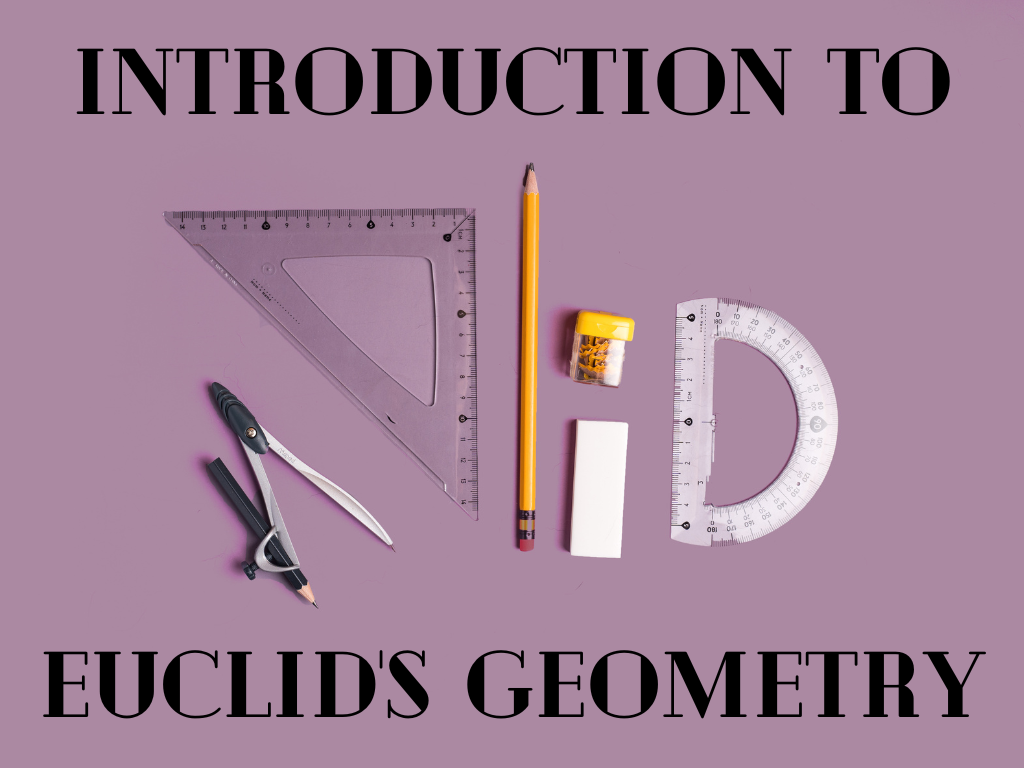## Introduction to Euclid’s Geometry Class 9th

Euclid’s Definitions, Axioms, and Postulates Euclid listed 23 definitions in book 1 of the ‘Elements’. A few of them are given below : Euclid gave some certain properties which were assumed by him and which were not proved. Actually, these assumptions were ‘obvious universal truths’. There were two types of these assumptions: axioms and postulates. …## The Similarity of Triangles Class 10th

Introduction The triangle is the shape, we are well known about it and its properties. We have studied different triangles and terms related to them like a scalene triangle, equilateral triangle, isosceles triangle, the area of the triangle, the perimeter of a triangle, the median of a triangle, the altitude of the triangle, etc. In …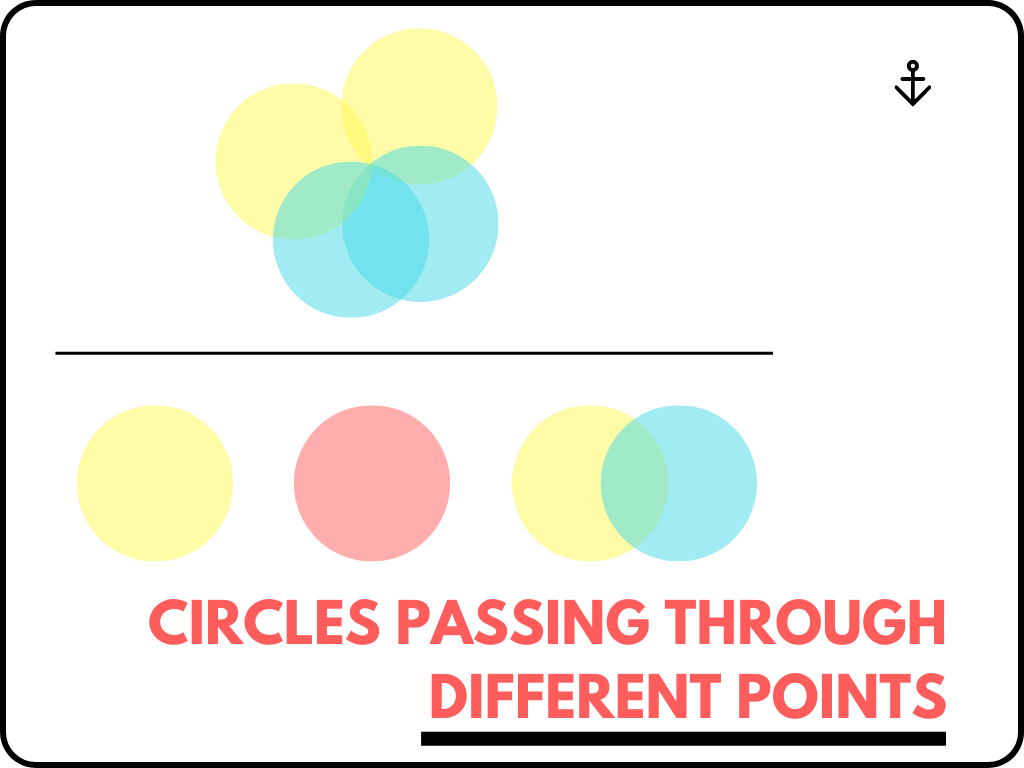## Circles Passing Through Different Points Class 10th

Circles Passing Through Different Points are as follows. Circles Passing Through One Point If there is a point in a plane then through that point many circles can pass. We can say infinite circles can pass. We can understand it practically from the figure given below. Circles Passing Through Two Points If there are two …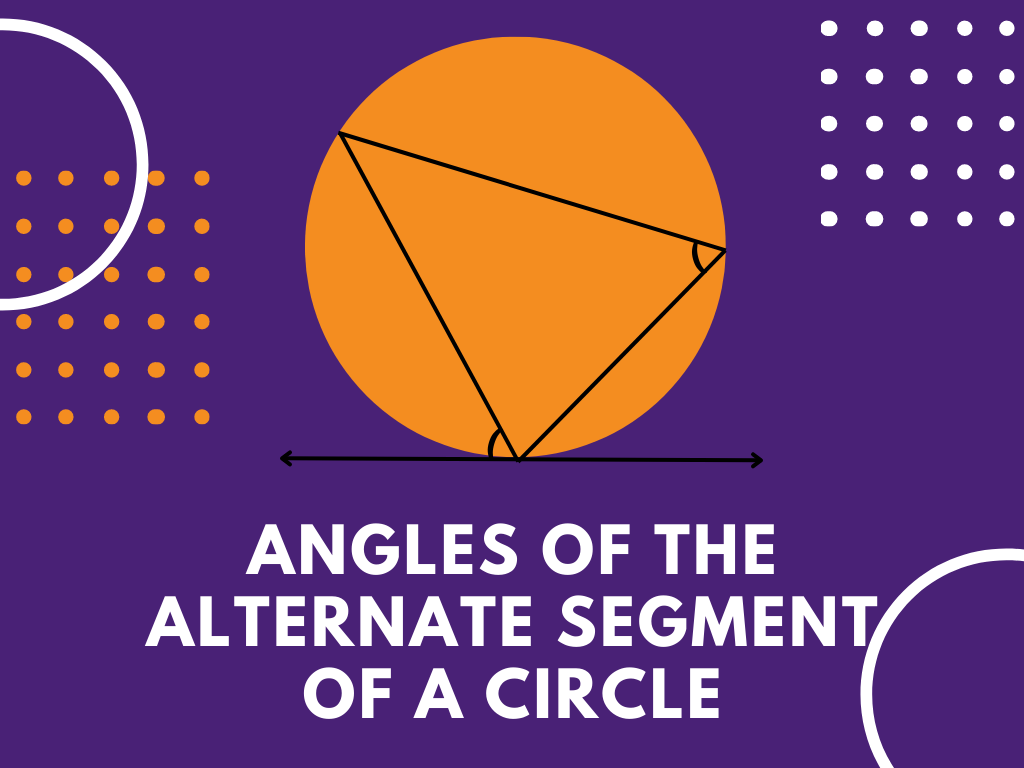## Angles of the Alternate Segment of a Circle Class 10th

Introduction In a circle, if a tangent is drawn then at the point of contact we draw a chord. This chord divides the circle into two segments then the angle made by the chord with the tangent will be equal to the angle made in the alternate segment by the chord. This is the concept …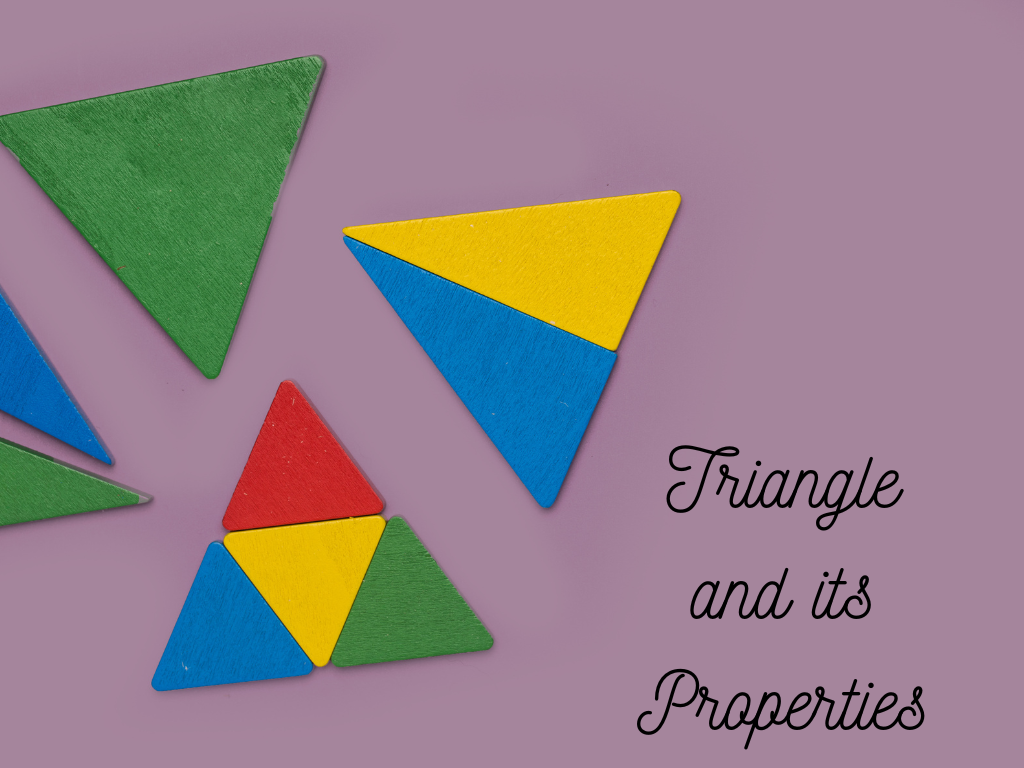## Triangle and its Properties Class 10th

Definition A Closed figure made of three line segments is called a Triangle. It has three sides, three angles, and three vertices. Here, Triangle ABC and its Properties are shown below. Three sides – AB, BC, CA Three angles – ∠A, ∠B, ∠C Three vertices – A, B, C Classification of Triangles – ➤ Based on …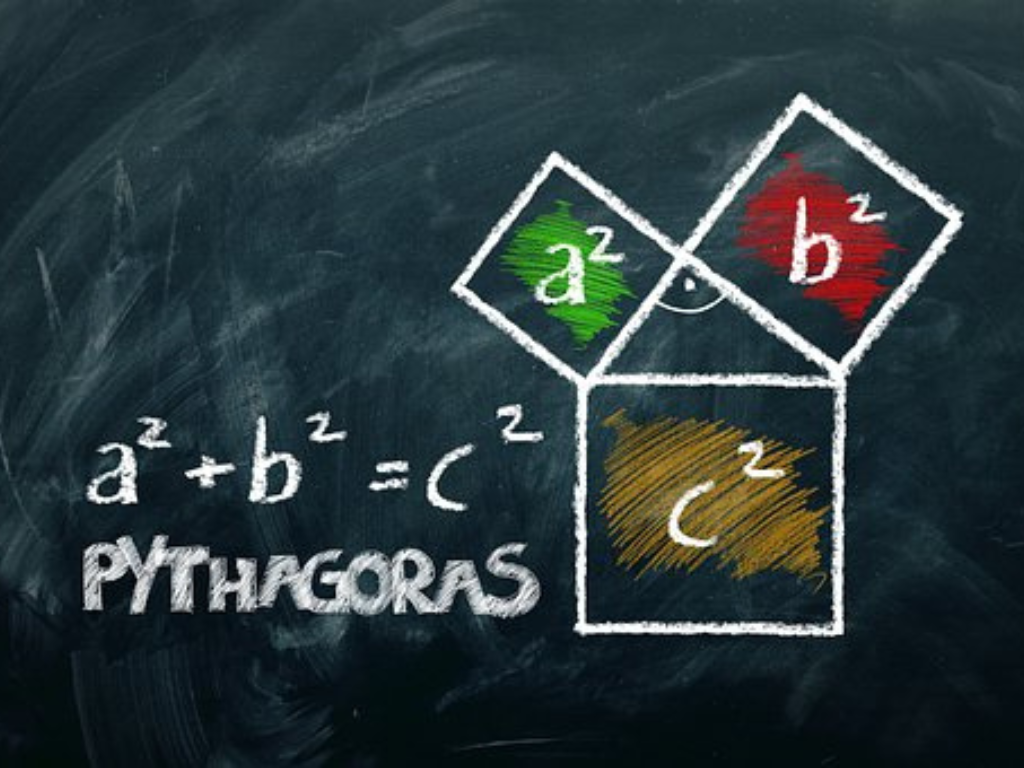## Pythagoras Theorem (Boudhayana Theorem) Class 10th

Introduction The Pythagoras theorem is given by the Greek mathematician Pythagoras. Before Pythagoras, this theorem was discovered by Indian mathematician Boudhayana so this theorem is also known as Boudhayana Theorem. This theorem is also called Pythagorean Theorem. Statement – According to this theorem, In a right-angled triangle, the square of the hypotenuse side is equal …